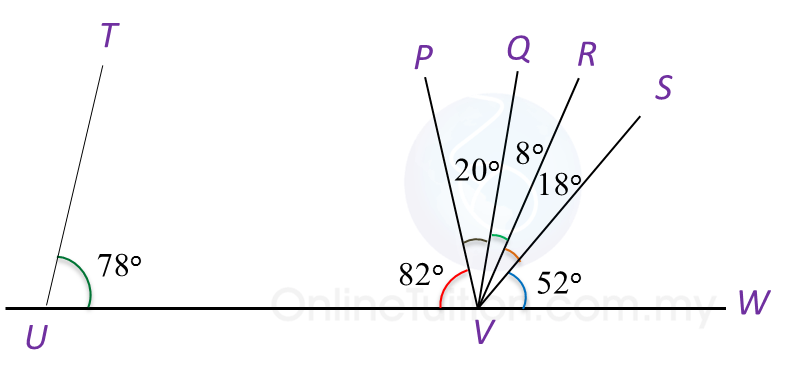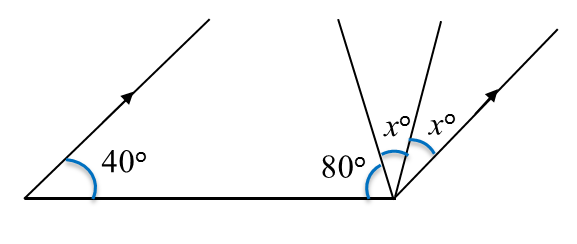# 8.2.3 Lines and Angles, PT3 Practice

Question 7:
In Diagram below, PWV is a straight line.(a) Which line is perpendicular to line PWV?
(b) State the value of  ∠ RWU.

Solution:
(a) SW

(b) ∠ RWU = 13o + 29o + 20o = 62o

Question 8:
In Diagram below, UVW is a straight line.(a) Which line is parallel to line TU?
(b) State the value of  ∠ QVS.

Solution:
(a) QV

(b) ∠ QVS = 8o + 18o = 26o

 
 
Question 9:
 
In Diagram below, find the value of x.(adsbygoogle = window.adsbygoogle || []).push({}); 
Solution:
 
40o + 80o + xo + xo = 180o
 
2xo = 180o – 120o 
 
2xo = 60o
 
x= 30o
 
x = 30
 
 
 
 
 
 
Question 10:
 
In Diagram below, PQR is an isosceles triangle and QRS is a straight line.Find the values of x and y.
 
 

  Solution:
 
  $\begin{array}{l}\angle PRQ={180}^{o}-{110}^{o}={70}^{o}\\ \angle PRQ=\angle PQR={70}^{o}\\ \text{}{y}^{o}={180}^{o}-{70}^{o}-{70}^{o}\\ \text{}={40}^{o}\\ \text{}y=40\\ {x}^{o}={110}^{o}-{40}^{o}\\ \text{}={70}^{o}\\ \text{}x=70\end{array}$